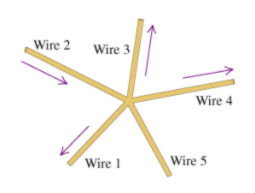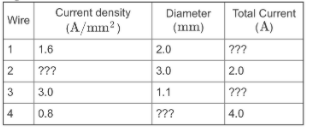# Problem: A Five Wire Junction Learning Goal: To learn to apply the concept of current density and Kirchhoff's junction rule. Consider a junction of five wires, as shown in the figure. The arrows indicate the direction of current flow. (Figure 1) The information about the magnitudes of the current density and the diameters for wires 1,2,3, and 4 is given in the table. Some of the values are unknown. Part A. Find the current I5 in wire 5. Express your answer in amperes. Use two significant figures. Assume that the current out of the junction is positive and that the current into the junction is negative.

###### FREE Expert Solution

Current density:

$\overline{){\mathbf{J}}{\mathbf{=}}\frac{\mathbf{i}}{\mathbf{A}}}$

96% (21 ratings)###### Problem Details

A Five Wire Junction Learning Goal: To learn to apply the concept of current density and Kirchhoff's junction rule.

Consider a junction of five wires, as shown in the figure. The arrows indicate the direction of current flow. (Figure 1)The information about the magnitudes of the current density and the diameters for wires 1,2,3, and 4 is given in the table. Some of the values are unknown.Part A. Find the current I5 in wire 5. Express your answer in amperes. Use two significant figures. Assume that the current out of the junction is positive and that the current into the junction is negative.